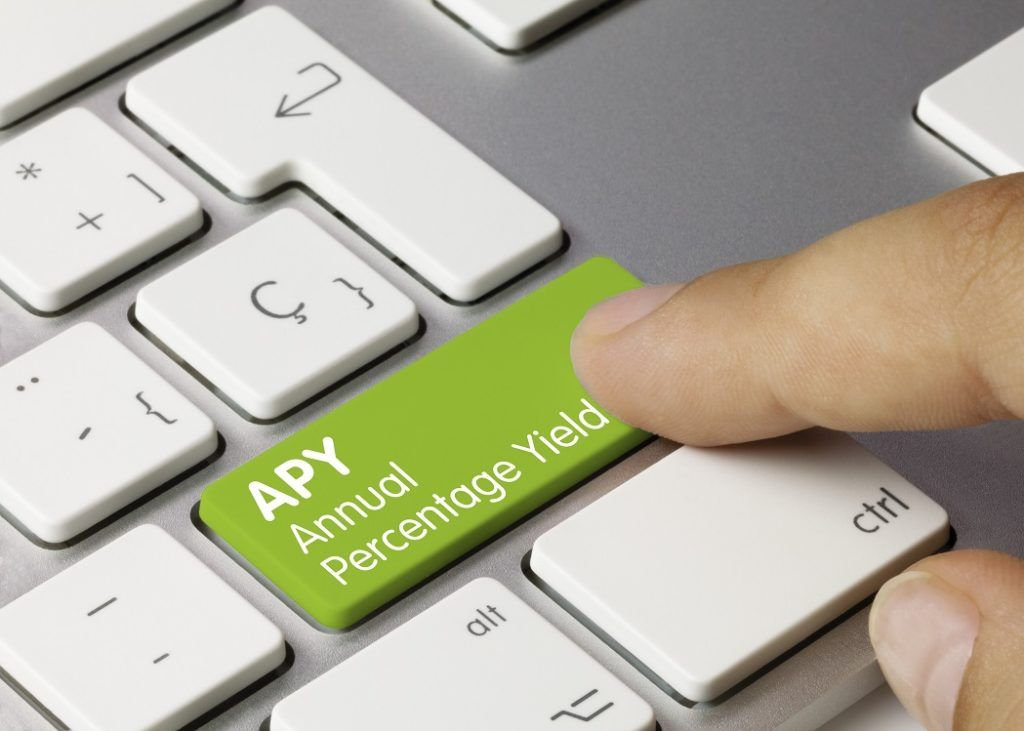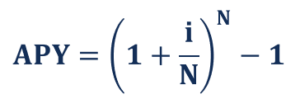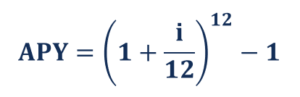# Annual Percentage Yield

A normalized interest rate based on the compounding period of one year

## What is the Annual Percentage Yield?

The annual percentage yield (APY) is a normalized interest rate based on the compounding period of one year. The APY provides a standardized representation of the underlying interest rates of financial products.The primary advantage of the annual percentage yield is the consideration of the compounding effect. Recall that compounding is essentially a process in which an asset or liability makes earnings and interests on both principal and capital gains or interest. In other words, it provides the true interest rate that a lender or an investor will earn.

Using the annual percentage yield, one can compare various financial products whose interest rates come with different compounding periods. However, the APY does not consider potential account fees that can affect the net gain.

### Formula for Annual Percentage Yield

The general formula to calculate the annual percentage yield (APY) is expressed using the following mathematical equation:Where:

• i – the nominal interest rate
• N – the number of compounding periods

For example, if the interest rate is compounded monthly, the relevant formula to calculate the annual percentage yield is the following:### APY vs. APR

Although both the annual percentage yield (APY) and annual percentage rate (APR) are representations of interest rate, there is a significant distinction between the two terms. Unlike the annual percentage yield, the annual percentage rate does not consider compounding effects and quotes the actual yearly cost of funds.

As mentioned above, the primary advantage of the APY over the APR is the standardized representation of interest rates. In other words, the former can be utilized to compare products with various compounding structures for interest rates.

Since the APY takes into consideration the compounding effect, it is higher than the APR. Due to such a reason, financial institutions tend to prefer to quote the APR in their loan offerings as the lower rate makes their deals more attractive to potential customers.

For the same reason, the annual percentage yield is frequently reported in the offerings of investment products or interest-earning bank accounts as investors are willing to receive higher interest rates on their investments.

### More Resources

CFI is the official provider of the global Financial Modeling & Valuation Analyst (FMVA)™ certification program, designed to help anyone become a world-class financial analyst. To keep advancing your career, the additional resources below will be useful:

• Continuously Compounded Return
• Effective Annual Interest Rate
• Simple Interest
• Rate of Return

### Financial Analyst Training

Get world-class financial training with CFI’s online certified financial analyst training program!

Gain the confidence you need to move up the ladder in a high powered corporate finance career path.

Learn financial modeling and valuation in Excel the easy way, with step-by-step training.